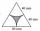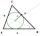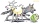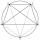Park

In the newly built park will be permanently placed a rotating sprayer irrigation of lawns. Determine the largest radius of the circle which can irrigate by sprayer P so not to spray park visitors on line AB. Distance AB = 55 m, AP = 36 m and BP = 28 m.

Result

r =  16.19 m

Solution:Try calculation via our triangle calculator.

Leave us a comment of example and its solution (i.e. if it is still somewhat unclear...):Be the first to comment!To solve this example are needed these knowledge from mathematics:

Do you want to convert length units? See also our trigonometric triangle calculator.

Next similar examples:

1. Center traverseIt is true that the middle traverse bisects the triangle?
2. The farmerThe farmer would like to first seed his small field. The required amount depends on the seed area. Field has a triangular shape. The farmer had fenced field, so he knows the lengths of the sides: 119, 111 and 90 meters. Find a suitable way to determine th
3. AceThe length of segment AB is 24 cm and the point M and N divided it into thirds. Calculate the circumference and area of this shape.
4. Circular lawnAround a circular lawn area is 2 m wide sidewalk. The outer edge of the sidewalk is curb whose width is 2 m. Curbstone and the inner side of the sidewalk together form a concentric circles. Calculate the area of the circular lawn and the result round to 1
5. Sss triangleCalculate the area and heights in the triangle ABC by sides a = 8cm, b = 11cm, c = 12cm
6. Equilateral triangle v3Calculate the content of the colored gray part. Equilateral triangle has side length 8 cm. Arc centers are the vertices of a triangle.
7. Circles 2Calculate the area bounded by the circumscribed and inscribed circle in triangle with sides 12 cm, 14 cm, 18 cm.
8. TriangleThe triangle has known all three sides: a=5.5 m, b=5.3 m, c= 7.8 m. Calculate area of ​this triangle.
9. Chord 2Point A has distance 13 cm from the center of the circle with radius r = 5 cm. Calculate the length of the chord connecting the points T1 and T2 of contact of tangents led from point A to the circle.
10. TriangleDetermine whether a triangle can be formed with the given side lengths. If so, use Heron's formula to find the area of the triangle. a = 158 b = 185 c = 201
11. TriangleCalculate heights of the triangle ABC if sides of the triangle are a=75, b=84 and c=33.
12. Inscribed circleThe circle inscribed in a triangle has a radius 3 cm. Express the area of the triangle using a, b, c.
13. DiamondRhombus has side 17 cm and and one of diagonal 22 cm long. Calculate its area.
14. An equilateralAn equilateral triangle with a side 10 m represents a wooden platform standing in a lawn. A goat is tied to a corner with a 15 m rope. What is the maximum amount of grazing area available to the goat?
15. SinesIn ▵ ABC, if sin(α)=0.5 and sin(β)=0.6 calculate sin(γ)
16. 10 piecesHow to divide the circle into 10 parts (geometrically)?
17. Theorem proveWe want to prove the sentence: If the natural number n is divisible by six, then n is divisible by three. From what assumption we started?